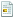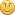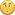September 26, 2022, 12:29:01 AM

## News:

IonicWind Snippit Manager 2.xx Released!  Install it on a memory stick and take it with you!  With or without IWBasic!

## Programming 5 - Data Types and Operations

Started by GWS, April 29, 2010, 06:14:38 AM

0 Members and 1 Guest are viewing this topic.

#### GWS

#####April 29, 2010, 06:14:38 AMLast Edit: April 29, 2010, 11:02:04 AM by GWS
Hi folks,

Here we go with the next section - using variables ..Programming 5.

Now that we have 'Variable' data quantities to hold the data for the application, we'll take a look at how we load and process the data.

To try out the ideas using Integer numbers, set up the following console program in the editor window.

`openconsoledef i, j as intprint : print "Press any key to exit"do: until inkey\$<>""closeconsoleend`

Here we are declaring two Integer variables 'i' and 'j'.

[Note the helpful statements to 'Press any key to exit'.  The first 'print' gives a new line, the' : ' means there is another statement following. In this case:  print "Press any key to exit"].

The simplest way to place data in a variable is just to program it in .. so we'll load the two Integers with the following statements ..
(Just type or copy and paste them into the program between the variable definitions and the 'Press any key to exit' line).

`i = 5j = 6print "i = ", i ,"   ", "j = ", j`

The print statement will report what the values are set to .. as you can see if you run the program.

So now we'll do something with the variables, and print the result, using the following statements ..

`i = i + jprintprint "The Sum of i and j = ", iprintprint "The new value of i = ", i`

The statement 'i = i + j' may appear strange - remember we mentioned earlier that a statement looks a lot like an equation - but it isn't quite the same.

Here's what happens when the statement i = i + j is executed.

The right-hand expression is evaluated first.  Currently i = 5, and j = 6, so we get 5 + 6 which is of course 11.

Then the result of the right-hand side is loaded into the memory reserved for variable 'i'.
So the original 'i' value of 5 is overwritten by the result of the expression 'i + j' and the new value of 'i' becomes 11.

It's a bit clumsy to express in words, but the result of the right-hand expression is placed in the left-hand variable - the original value is lost.

[Note that the print instructions contain descriptive text, as well as printing the values themselves].

If you run the program now, you will see the new value of 'i' is 11, and this is indeed the total.

We could have used a third integer variable 'k' to hold the result, if we didn't want to lose the original 'i' value.
I chose to use just the two variables to illustrate how a statement works.

Now that the program is working, let's play with it a bit.  Change the description text of the result to suit the calculation.

Try changing the the addition to subtraction :   i = i - j      The result will be  -1.

Now try multipication:   i = i * j     The result will be 30.

Finally try division.       i = i / j      And the result is ....??

I expect that surprised you.   Zero !!

Well, five divided by six is less than one ( 0.83 as a decimal ).
But our left-hand variable 'i' is only capable of holding integer whole numbers.
Consequently, the decimal 0.83 result is truncated and 'i' finishes up as just the whole number Zero.

Oh look! I'm suggesting the expression value 0.83 is in there somewhere, even though both variables are integers.

Exactly so - Creative has this great feature, that ALL your calculations are actually done in double precision decimal arithmetic. This is the most accurate type of decimal arithmetic (to 15 significant figures).  The result is then adjusted to fit the type of left-hand variable - in this case an integer.  So all we get from a very precise calculation is Zero.

Want to prove it?  Just replace the definitions block with these definitions so we define 'i' as a double precision quantity ..

`def j as intdef i as double`

Run the program again, and you will now get the answer 0.83.

The mathematicians among you might say 'that's not very accurate - 5 / 6 = 0.8333333333333'.

Oh, all right then, I'll admit we missed a statement out.  Try putting the statement 'setprecision 15' after the definitions block, and run it again.

That's more like it.   Creative only presents results to 2 decimal places by default.  If you prefer to see more, just use a 'setprecision' statement.

Remember though, that calculations are always done to maximum accuracy in the background.

Instead of loading variables directly, a more common situation is when you obtain values from the user.

To do this, simply include a statement like :

`input "Please enter a value for i  : ", i`

instead of the specific setting 'i = 5' we just used.

___________________________________________________

Next we''ll have a look at Decimal numbers.  Close the previous program, and copy and paste this one ..

`openconsoledef x, y, z as float setprecision 10x = 5.0y = 3.303print "x = ", x ,"   ", "y = ", yz = x + yprintprint "The Sum of x and y = ",zprint:print "Press any key to exit,"do: until inkey\$<>""closeconsoleend`

If you run this program, the results will again surprise you.

The value of 'x' is 5 alright, but the value of 'y' and the result look very odd.

Decimal numbers are tricky, and have to be handled with care. Even if you increase the
ÃƒÂ¢Ã¢â€šÂ¬Ã‹Å"setprecisionÃƒÂ¢Ã¢â€šÂ¬Ã¢â€žÂ¢ statement value to 15 decimal places, you will still not get the correct answer, which is 8.3030000000.

So what's the problem ?    Well variables of type 'Float' can only represent up to 7 significant figures.  Any additional digits will not be accurate.

In our example, the variable 'y' should hold the value 3.303, but the ability of a 'float' type to represent a decimal quantity is limited.
After the seventh digit, the digits are not precise.

So we see 'y' = 8.302999, followed by the inaccurate digits 4965.  The 'error' is in fact only 1 part in a million - which is pretty accurate - but it does look strange.

You are encountering a disturbing feature of decimal numbers called ÃƒÂ¢Ã¢â€šÂ¬Ã‹Å"precisionÃƒÂ¢Ã¢â€šÂ¬Ã¢â€žÂ¢.

To correct the matter, change the definition of the variables from 'Float' to 'Double'.

We have now defined the working variables as ÃƒÂ¢Ã¢â€šÂ¬Ã‹Å"doubleÃƒÂ¢Ã¢â€šÂ¬Ã¢â€žÂ¢ precision type, and they are now accurate to 16 significant figures.

Run the program again, and all is now correct.

Normally, most mathematical calculations involving decimals will give accurate answers if you use ÃƒÂ¢Ã¢â€šÂ¬Ã‹Å"doubleÃƒÂ¢Ã¢â€šÂ¬Ã¢â€žÂ¢ precision variables.
However, if you need to carry out lengthy, high-precision calculations, always be on the lookout for loss of precision.

If you are happy with ÃƒÂ¢Ã¢â€šÂ¬Ã‹Å"slide ruleÃƒÂ¢Ã¢â€šÂ¬Ã¢â€žÂ¢ accuracy, the ÃƒÂ¢Ã¢â€šÂ¬Ã‹Å"FloatÃƒÂ¢Ã¢â€šÂ¬Ã¢â€žÂ¢ type variable will be more than sufficient.

You can try out changing the operation from Addition, to Subtract, Multiply and Divide.

____________________________________________________

Finally in this section, we will check out String operations.

Close the previous program and copy and paste this one ..

`openconsoledef a\$, b\$ as Stringa\$ = "Donald duck"b\$ = "Minnie mouse"print a\$, b\$print:print "Press any key to exit,"do: until inkey\$<>""closeconsoleend`

Here we are setting the value of a\$ to a string of characters "Donald duck", and b\$ to another string of characters "Minnie mouse".

Dating back to the days of home computing, I got used to placing a '\$' symbol after string variable names to identify them clearly.
You don't need to do this - it just makes your string variables easier to recognise.

Note that strings are enclosed in quotes.

When you run this program, you get the result: 'Donald DuckMinnie mouse'.  The two strings are just printed one after the other with no space between them.

Try changing the print instruction to print a\$ + b\$.  You will get the same result.
With strings, the '+' operation will append the second string to the first - a sort of 'addition'.

But we still need a space of some sort to look neat, so try this ..

print a\$,"   ", b\$

If you run the program, there is now a space - but another improvement would be:

print a\$," and ", b\$    or  print a\$ + " and " + b\$  whichever you prefer.

Strings are limited to 254 ASCII characters.  This is sufficient for most purposes.

There is another type of string variable - an 'Istring', but we'll leave discussion of those for a little while.

So can we write a 'subtraction' operation in the same way that we had the 'addiiton' of two strings?  Er .. no.
You would have to write a bit of code of your own to do that job.

Can we load a string of characters into an Integer or Float variable ?  Again no.  Characters are quite different from numeric values.

Can we load integer or decimal numbers into a string variable?  Not directly, but you can use the Str\$() function to do something of the kind.

So you might have a statement :

a\$ = Str\$(345.2)

The resulting contents of a\$ would be "345.2".   It looks the same, but these are just the string of characters "3 4 5 . 2".   You can not do any calculations with string quantities.

You can convert the string back into a number again using the Val() function.

End of Programming 5

_________________________________________________________________

Tomorrow may be too late ..